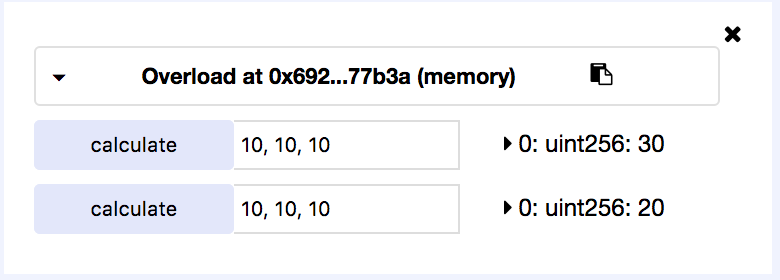Solidity does not support optional parameters, but what it does support is method overrides. Just like in Java you can declare a function multiple times as long as the arity and/or types are different.

Example I tried on remix:

``````pragma solidity ^0.4.18;

function calculate (uint _a, uint _b) public pure returns (uint) {
return _a + _b;
}

function calculate (uint _a, uint _b, uint _c) public pure returns (uint) {
return _a + _b + _c;
}
}
``````

Here's a silly example with an internal function that both use, just to demonstrate reusable functionality:

``````pragma solidity ^0.4.18;

function calculate (uint _a, uint _b) public pure returns (uint) {